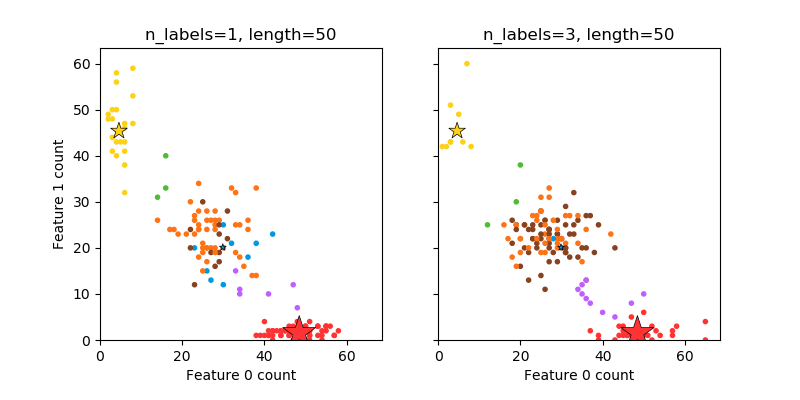/scikit-learn

# Plot randomly generated multilabel dataset

This illustrates the `datasets.make_multilabel_classification` dataset generator. Each sample consists of counts of two features (up to 50 in total), which are differently distributed in each of two classes.

Points are labeled as follows, where Y means the class is present:

1 2 3 Color
Y N N Red
N Y N Blue
N N Y Yellow
Y Y N Purple
Y N Y Orange
Y Y N Green
Y Y Y Brown

A star marks the expected sample for each class; its size reflects the probability of selecting that class label.

The left and right examples highlight the `n_labels` parameter: more of the samples in the right plot have 2 or 3 labels.

Note that this two-dimensional example is very degenerate: generally the number of features would be much greater than the “document length”, while here we have much larger documents than vocabulary. Similarly, with `n_classes > n_features`, it is much less likely that a feature distinguishes a particular class.Out:

```The data was generated from (random_state=1013):
Class   P(C)    P(w0|C) P(w1|C)
red     0.64    0.97    0.03
blue    0.06    0.60    0.40
yellow  0.30    0.09    0.91
```
```from __future__ import print_function
import numpy as np
import matplotlib.pyplot as plt

from sklearn.datasets import make_multilabel_classification as make_ml_clf

print(__doc__)

COLORS = np.array(['!',
'#FF3333',  # red
'#0198E1',  # blue
'#BF5FFF',  # purple
'#FCD116',  # yellow
'#FF7216',  # orange
'#4DBD33',  # green
'#87421F'   # brown
])

# Use same random seed for multiple calls to make_multilabel_classification to
# ensure same distributions
RANDOM_SEED = np.random.randint(2 ** 10)

def plot_2d(ax, n_labels=1, n_classes=3, length=50):
X, Y, p_c, p_w_c = make_ml_clf(n_samples=150, n_features=2,
n_classes=n_classes, n_labels=n_labels,
length=length, allow_unlabeled=False,
return_distributions=True,
random_state=RANDOM_SEED)

ax.scatter(X[:, 0], X[:, 1], color=COLORS.take((Y * [1, 2, 4]
).sum(axis=1)),
marker='.')
ax.scatter(p_w_c * length, p_w_c * length,
marker='*', linewidth=.5, edgecolor='black',
s=20 + 1500 * p_c ** 2,
color=COLORS.take([1, 2, 4]))
ax.set_xlabel('Feature 0 count')
return p_c, p_w_c

_, (ax1, ax2) = plt.subplots(1, 2, sharex='row', sharey='row', figsize=(8, 4))

p_c, p_w_c = plot_2d(ax1, n_labels=1)
ax1.set_title('n_labels=1, length=50')
ax1.set_ylabel('Feature 1 count')

plot_2d(ax2, n_labels=3)
ax2.set_title('n_labels=3, length=50')
ax2.set_xlim(left=0, auto=True)
ax2.set_ylim(bottom=0, auto=True)

plt.show()

print('The data was generated from (random_state=%d):' % RANDOM_SEED)
print('Class', 'P(C)', 'P(w0|C)', 'P(w1|C)', sep='\t')
for k, p, p_w in zip(['red', 'blue', 'yellow'], p_c, p_w_c.T):
print('%s\t%0.2f\t%0.2f\t%0.2f' % (k, p, p_w, p_w))
```

Total running time of the script: ( 0 minutes 0.084 seconds)

Gallery generated by Sphinx-Gallery